# Module 3 Exam Review

 Module 3 Exam Review This review is neither a replica of the exam nor does it contain every concept in the module. It covers just the material from the regular lessons. It is always best practice to review all lessons and assessments. Resubmitting is a great way to review/learn and improve your grade. In this review, questions 1 – 32 are the general topics for the multiple choice type questions on the module exam; questions 33 – 37 are covered as essays on the exam. There are approximately 20 questions (about 3 are essays) on the regular exam; approximately 25 questions (about 6 are essays) on the honors exam. The number in parenthesis is the lesson. None of the honors lessons are in this review. The answer key is after the review. Be sure to have a periodic table with the chemical symbol and name for the review and the exam. Do you need extra help or have a question about a question? Join our live 1-on1 help sessions. The current schedule and link to join is on the course announcement page. Multiple Choice Which of the following did Mosley believe? (3.01) The elements should be arranged based upon current knowledge of electrons. The elements should be arranged based upon the number of neutrons. The periodic table should be organized based upon atomic number. The periodic table should be organized based upon chemical properties. What is significant about elements in the same group on the periodic table? (3.02) They have the same number of protons. They have the same number of electrons. They have the similar chemical properties. They are all noble gases. As you go left to right across the periodic table, which of the following is true? (3.02) The elements have the same number of valence electrons. The elements increase in radius. The elements have the same common charge. The elements increase the number of protons. Chlorine (Cl), Oxygen (O), Xenon (Xe) and Bromine (Br) are all nonmetals. Using the periodic table, which pair of elements has the most similar properties? (3.02) Chlorine and oxygen Oxygen and xenon Xenon and hydrogen Bromine and chlorine Using the periodic table, which of the following elements has the LEAST properties in common with Oxygen (O)? (3.02) Selenium Iodine Polonium Sulfur Which of the following pairs of elements could possibly be in the same group? (3.02) X has a 3+ ion; Y has a 3- ion. X tends to form a 1+ ion; Y has one valence electron. X will tend to gain two electrons; Y has two valence electrons. X has an atomic number 49; Y forms a 2+ ion. Using the periodic table, which of the following elements has the lowest electronegativity value? (3.03) Calcium Cesium Nitrogen Fluorine Which of the following elements has the smallest radius? (3.03) Carbon Rubidium Radon Neon Which of the following elements has the greatest first ionization energy? (3.03) Sulfur Neon Helium Lithium The valence electrons of the substance's atoms feel an effective nuclear charge of +5. If the unknown substance has higher ionization energies than gallium (Ga), what other characteristics would you expect the substance to exhibit? (3.03) It would have smaller atomic radii than Ga and a higher electronegativity value than Ga. It would have larger atomic radii than Ga and a higher electronegativity value than Ga. It would have smaller atomic radii than Ga and a lower electronegativity value than Ga. It would have smaller atomic radii than Ga and a lower electronegativity value than Ga. Which of the following atoms has 3 valence electrons? (3.05) Aluminum Carbon Nitrogen Xenon Which of the following atoms has 4 valance electrons? (3.05) Hydrogen Carbon Nitrogen Potassium When one atom of lithium (Li) combines with one atom of chlorine (Cl), their bonding results in a molecule of LiCl. Which of the following describes the arrangement of valence electrons in a bond between Li and Cl? (3.05) The valence electrons spend more time around Li The valence electrons are given up by the Li and gained by the Cl The valence electrons are shared equally between Li and Cl These two atoms will not form a bond. Which of the following would form an ionic bond when combined? (3.05) Hydrogen and helium Carbon and hydrogen Aluminum and oxygen Aluminum and iron What is the formula of a compound formed between magnesium and oxygen? (3.05) MgO Mg2O2 MgO2 OMg Which of the following is not a correct formula unit? (3.05) AlP NaF CaS2 HF Which of the following is NOT a diatomic element found in nature? (3.06) H2 P2 Cl2 Br2 Do all diatomic elements have only a single bond? (3.06) Yes No Which of the following is the correct description of an ionic bond? (3.04) Electrons are shared between atoms so that stable electron configurations are achieved An ionic bond exists between elements with large electronegativity differences An ionic bond exists between two nonmetals An ionic bond experiences very small differences in electronegativity values Which of the following Lewis dot structures is correct for the molecule that forms when nitrogen bonds with fluorine? (3.06)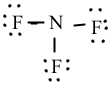b.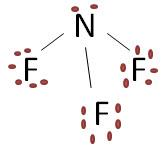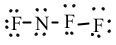d.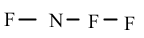As a covalent bond lengthens, what happens to its’ strength? (3.06) The strength increases. The strength decreases. The strength of a bond is not measureable. There is no change to the strength of a bond. What is a correct name for H2SO4? (3.08) Hydrogen sulfide Hydrogen sulfite Hydrogen sulfate Hydrogen sucrate What is another correct name for H2SO4 if we were to name it as an acid? (3.08) It cannot be named as an acid Sulfuric acid Sulfurous acid Hydrosulfuric acid What is the correct name for Pb3(PO4)2? (3.08) Lead phosphide Lead phosphate Lead (II) phosphate Lead (III) phosphate What is the correct formula when aluminum bonds with hydroxide? (3.08) AlOH OHAl Al(OH)3 Al(OH)2 What is the correct formula for potassium chlorate? (3.08) PClO3 KClO3 PCl K(ClO3)2 How many atoms of copper are in one formula unit of copper (II) sulfate? (3.08) One Two Three Four Which of the following is NOT true about hydrogen bonding? (3.07) It is an intermolecular force It exists between any element and hydrogen. It is the strongest of all the intermolecular forces. Water experiences hydrogen bonding. Electrostatic attraction of permanent dipoles in polar molecules is a definition of which type of intermolecular force? (3.07) London Dispersion Dipole-dipole Ion-Dipole Hydrogen Bonding Which of the following is true of London Dispersion forces? (3.07) The get weaker as the number of electrons increases. They are a permanent attractive force between two adjacent molecules forming permanent dipoles. They occur between all particles and molecules. They are stronger than hydrogen bonding. Dipole-dipole interactions form between which types of molecules? (3.07) Non-polar molecules Polar molecules Ionic compounds All of the above Which of the following does NOT share the same empirical formula? (3.09) H2O and H2O2 C4H16 and C2H8 C3H6O3 and C2H4O2 CH2 and C10H20 Essays Give the trend for electronegativity across a period and down a group, and explain each of these trends in terms of attraction, repulsion, and effective nuclear charge. (3.03) Why is the fourth ionization energy for Boron (B) much higher than its third ionization energy. Explain your answer in terms of attraction, repulsion, and electron arrangement. (3.03) Compare and contrast the compounds H2O and CO2 in terms of covalent bonding, molecular geometry (shape), and intermolecular forces. Which substance would experience a stronger attraction between its molecules, and why? (3.06, 3.07) Consider the compound NH4Cl and answer the following questions. (3.08, 3.09) What is the name of this compound? What is the molar mass of this compound? Explain how you calculated this value. Consider the compound FePO4 and answer the following questions. (3.08, 3.09) What is the name of this compound? What is the molar mass of this compound? Explain how you calculated this value. Module 3 Exam Review ANSWER KEY Multiple Choice Which of the following did Mosley believe?(3.01) The elements should be arranged based upon current knowledge of electrons. The elements should be arranged based upon the number of neutrons. The periodic table should be organized based upon atomic number. The periodic table should be organized based upon chemical properties. What is significant about elements in the same group on the periodic table? (3.02) They have the same number of protons. They have the same number of electrons. They have the similar chemical properties. They are all noble gases. As you go left to right across the periodic table, which of the following is true? (3.02) The elements have the same number of valence electrons. The elements increase in radius. The elements have the same common charge. The elements increase the number of protons. Chlorine (Cl), Oxygen (O), Xenon (Xe) and Bromine (Br) are all nonmetals. Using the periodic table, which pair of elements has the most similar properties? (3.02) Chlorine and oxygen Oxygen and xenon Xenon and hydrogen Bromine and chlorine Using the periodic table, which of the following elements has the LEAST properties in common with Oxygen (O)? (3.02) Selenium Iodine Polonium Sulfur Which of the following pairs of elements could possibly be in the same group? (3.02) X has a 3+ ion; Y has a 3- ion. X tends to form a 1+ ion; Y has one valence electron. X will tend to gain two electrons; Y has two valence electrons. X has an atomic number 49; Y forms a 2+ ion. Using the periodic table, which of the following elements has the lowest electronegativity value? (3.03) Calcium Cesium Nitrogen Fluorine Which of the following elements has the smallest radius? (3.03) Carbon Rubidium Radon Neon Which of the following elements has the greatest first ionization energy? (3.03) Sulfur Neon Helium Lithium The valence electrons of the substance's atoms feel an effective nuclear charge of +5. If the unknown substance has higher ionization energies than gallium (Ga), what other characteristics would you expect the substance to exhibit? (3.03) It would have smaller atomic radii than Ga and a higher electronegativity value than Ga. It would have larger atomic radii than Ga and a higher electronegativity value than Ga. It would have smaller atomic radii than Ga and a lower electronegativity value than Ga. It would have smaller atomic radii than Ga and a lower electronegativity value than Ga. Which of the following atoms has 3 valence electrons? (3.05) Aluminum Carbon Nitrogen Xenon Which of the following atoms has 4 valance electrons? (3.05) Hydrogen Carbon Nitrogen Potassium When one atom of lithium (Li) combines with one atom of chlorine (Cl), their bonding results in a molecule of LiCl. Which of the following describes the arrangement of valence electrons in a bond between Li and Cl? (3.05) The valence electrons spend more time around Li The valence electrons are given up by the Li and gained by the Cl The valence electrons are shared equally between Li and Cl These two atoms will not form a bond. Which of the following would form an ionic bond when combined? (3.05) Hydrogen and helium Carbon and hydrogen Aluminum and oxygen Aluminum and iron What is the formula of a compound formed between magnesium and oxygen? (3.05) MgO Mg2O2 MgO2 OMg Which of the following is not a correct formula unit? (3.05) AlP NaF CaS2 HF Which of the following is NOT a diatomic element found in nature? (3.06) H2 P2 Cl2 Br2 Do all diatomic elements have a single bond? (3.06) Yes No Which of the following is the correct description of an ionic bond? (3.04) Electrons are shared between atoms so that stable electron configurations are achieved An ionic bond exists between elements with large electronegativity differences An ionic bond exists between two nonmetals An ionic bond experiences very small differences in electronegativity values Which of the following Lewis dot structures is correct for the molecule that forms when nitrogen bonds with fluorine? (3.06)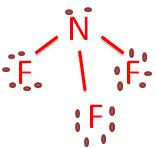a. b.d.As a covalent bond lengthens, what happens to its’ strength? (3.06) The strength increases. The strength decreases. The strength of a bond is not measureable. There is no change to the strength of a bond. What is a correct name for H2SO4? (3.08) Hydrogen sulfide Hydrogen sulfite Hydrogen sulfate Hydrogen sucrate What is another correct name for H2SO4 if we were to name it as an acid? (3.08) It cannot be named as an acid Sulfuric acid Sulfurous acid Hydrosulfuric acid What is the correct name for Pb3(PO4)2? (3.08) Lead phosphide Lead phosphate Lead (II) phosphate Lead (III) phosphate What is the correct formula when aluminum bonds with hydroxide? (3.08) AlOH OHAl Al(OH)3 Al(OH)2 What is the correct formula for potassium chlorate? (3.08) PClO3 KClO3 PCl K(ClO3)2 How many atoms of copper are in one formula unit of copper (II) sulfate? (3.08) One Two Three Four Which of the following is NOT true about hydrogen bonding? (3.07) It is an intermolecular force It exists between any element and hydrogen. It is the strongest of all the intermolecular forces. Water experiences hydrogen bonding. Electrostatic attraction of permanent dipoles in polar molecules is a definition of which type of intermolecular force? (3.07) London Dispersion Dipole-dipole Ion-Dipole Hydrogen Bonding Which of the following is true of London Dispersion forces? (3.07) The get weaker as the number of electrons increases. They are a permanent attractive force between two adjacent molecules forming permanent dipoles. They occur between all particles and molecules. They are stronger than hydrogen bonding. Dipole-dipole interactions form between which types of molecules? (3.07) Non-polar molecules Polar molecules Ionic compounds All of the above Which of the following does NOT share the same empirical formula? (3.09) H2O and H2O2 C4H16 and C2H8 C3H6O3 and C2H4O2 CH2 and C10H20 Essays Give the trend for electronegativity across a period and down a group, and explain each of these trends in terms of attraction, repulsion, and effective nuclear charge. (3.03) As you go left to right across the periodic table the effective nuclear charge increases. This means that the valence electrons are being held more tightly to the nucleus. Therefore, as you go left to right across the table, electronegativity increases. The greater an atom attracts its own valence electrons, the greater it is able to attract another atom’s electrons in a chemical bond. This also causes the radius to decrease. As you go down a group, the valence electrons are further from the nucleus, in higher energy levels. The electronegativity will decrease as you do down a group due to the increased distance. This also causes the atomic radius to increase. Why is the fourth ionization energy for Boron (B) much higher than its third ionization energy. Explain your answer in terms of attraction, repulsion, and electron arrangement. (3.03) Boron has 3 valence electrons in the 2nd energy level. The ionization energy for these three electrons is relatively low. Once those three electrons are removed, the 2nd energy level is empty. The fourth electron must come from the full 2s orbital. This inner electron has a greater attraction to the nucleus and is held more tightly. This causes the ionization energy of the 4th electrons to be significantly. Compare and contrast the compounds H2O and CO2 in terms of covalent bonding, molecular geometry (shape), and intermolecular forces. Which substance would experience a stronger attraction between its molecules, and why? (3.06, 3.07) Water is a polar covalent molecule which experiences a bent shape and hydrogen bonding. Carbon dioxide is a linear molecule, nonpolar and experiences London dispersion forces. Water would experience a stronger attraction between its molecules due to the hydrogen bonds. They are the strongest intermolecular force whereas London dispersion is the weakest. Consider the compound NH4Cl and answer the following questions. (3.08, 3.09) What is the name of this compound? This is ammonium chloride What is the molar mass of this compound? Explain how you calculated this value. N = 14.0067 X 1 = 14.0067 H = 1.00794 X 4 = 4.03176 Cl = 35.453 X 1 = 35.453 No add them: 14.0067 + 4.03176+ 35.453 = 53.49 g/mol Consider the compound FePO4 and answer the following questions. (3.08, 3.09) What is the name of this compound? We have Fe+? PO4-3 We know that Fe is positive since it’s a metal, but we do not know the charge. Since we have one of each, the charge of Fe has to be 3+. It is a transition metal, so we must have the Roman numeral indicating its charge in the name. This is Iron (III) phosphate. What is the molar mass of this compound? Explain how you calculated this value. We need the periodic table to get the molar masses: Fe = 55.845 X 1 = 55.845 P = 30.97 X 1 = 30.97 O = 16 X 3 = 48 Now add them: 55.845 + 30.97 + 16 = 102.82 g/molDownload 157.4 Kb.Share with your friends: